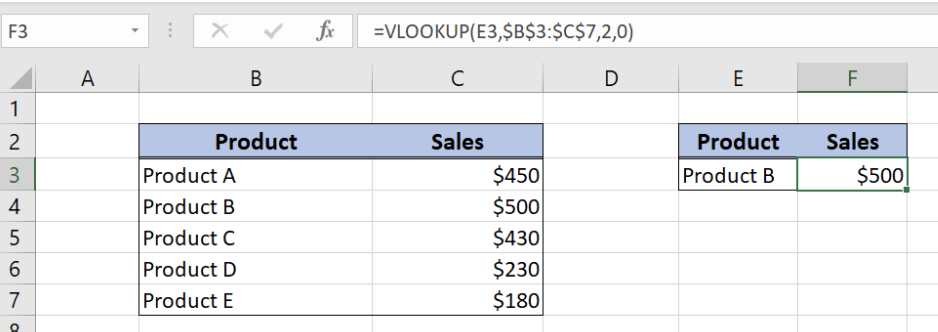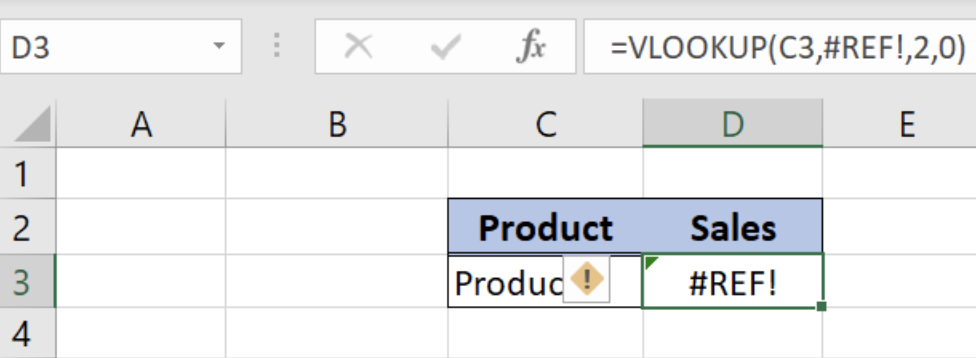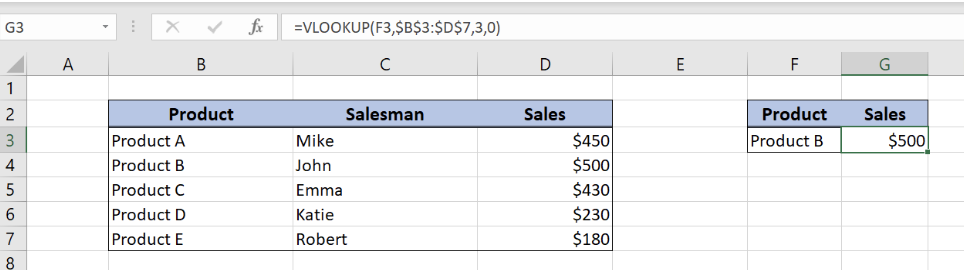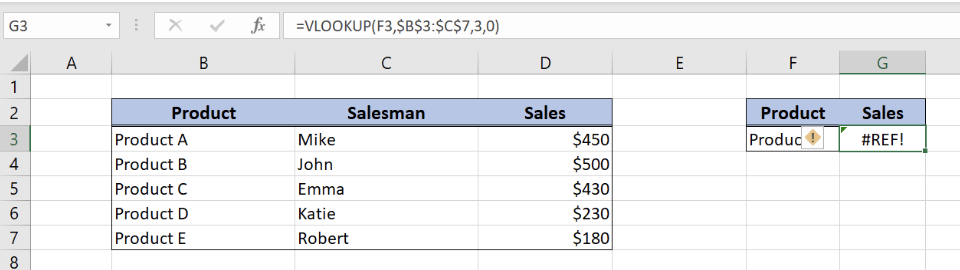Get instant live expert help with Excel or Google Sheets“My Excelchat expert helped me in less than 20 minutes, saving me what would have been 5 hours of work!”

#### Post your problem and you’ll get Expert help in seconds.

Your message must be at least 40 characters
Our professional Expert are available now. Your privacy is guaranteed.

# What to Do if You’re Getting a #REF Error with VLOOKUP

The VLOOKUP is one of the most popular functions in Excel. However, there can be many errors while using this function. In this tutorial, we will learn what to do if you’re getting a #REF! error with VLOOKUP. This error appears when data is missing or when we use a wrong table in the function. We will explain in detail and with examples both cases.

## #REF! Error because VLOOKUP table is missing

#REF! Error can appear if we have deleted a table in which we are looking up for the data. It can also appear if we have deleted a Worksheet or a Workbook which contains this table.

Let’s first look at the working example of the VLOOKUP function.Figure 1. The working example of the VLOOKUP function

As we can see in the picture, we have VLOOKUP formula in the cell F3. The purpose is to get the Sales value for Product B from the table in the range B2:C7. Our lookup columns is “Product” column. As the Sales is \$500, we got that value in the cell F3.

Now we will see what happens if we delete the table in which we’re looking up (B2:C7).Figure 2. The #REF! Error of the VLOOKUP function

In this example, we deleted columns containing our VLOOKUP table (B2:C7), but the formula remained the same. Now the formula from the cell F3 moved to the cell D3 as we deleted two columns. As you can see we got #REF! Error, as the formula didn’t manage to find the lookup table. Also, if we look at the formula, we will see that table is no longer \$B3:\$C\$7, instead #REF!

Now we know what to check first when we get #REF! Error. This cause is especially often when we are referencing a table in another worksheet or workbook. If someone deletes them, we will get this error.

## #REF! Error because VLOOKUP table is incomplete

#REF! Error can also appear if passed an incomplete table as a parameter to the VLOOKUP function. Usually, a user omits a column which needs to be retrieved.

We will again first look at the working example of the VLOOKUP function:Figure 3. The working example of the VLOOKUP function

Similarly to the first example, we have VLOOKUP formula in the cell G3. The purpose is to get the Sales value for Product B from the table in the range B2:D7. As the Sales is \$500, we got that value in the cell G3.

Now we will see what happens if we pass table B2:C7 by mistake, instead of B2:D7. If we do that, we omit the column which value we want to retrieve (D).Figure 4. The #REF! Error of the VLOOKUP function

In this example, in the VLOOKUP formula, we put table \$B\$3:\$C\$7, instead of \$B\$3:\$D\$7. As we want to get the value from the third column of the table (“Sales”), which we omitted, the VLOOKUP function won’t be able to work. The output of the function is #REF! Error.

As we can see from the previous explanation, this is the second most possible cause of the error. We should check if we have selected the whole table in the VLOOKUP function.

Most of the time, the problem you will need to solve will be more complex than a simple application of a formula or function. If you want to save hours of research and frustration, try our live Excelchat service! Our Excel Experts are available 24/7 to answer any Excel question you may have. We guarantee a connection within 30 seconds and a customized solution within 20 minutes.

Are you still looking for help with the VLOOKUP function? View our comprehensive round-up of VLOOKUP function tutorials here.

### Did this post not answer your question? Get a solution from connecting with the expert.Another blog reader asked this question today on Excelchat:
Solution examplesI have a pivot table in which per order (on the rows) stands how much products they ordered per size (on the columns). I want to determine which combinations of quantities of sizes people order. And I want to count these combinations.
Solved by E. W. in 60 minsI have 500 numbers in column A with aproximatly 15digits. In column B I have 5 numbers with 6 digits Can a find a formula wich can transfer all numbers from A column wich start with some 6 digitsa from B column
Solved by V. L. in 32 minsI have a sheet with 3 columns. First column is a code for records in column B (has 563 records). What I need is a formula to show me if what is in column C (has 4400 records) is in column B and if it is to take the code for that record. Example: Column A(code corresponding to column B): 12, 14, 15, 19 Column B(names): asd, adf, ade, aqw Column C(names): akd, adf, ade, anb, wgs I need something that would say, if record in column C (for example adf) is matching record in column B (adf) return the code from column A (the code corresponding to that record in column B),if not return blank.
Solved by C. J. in 8 minsIf the data in A matches the data in C, then I need the data in E to be placed in B
Solved by X. W. in 40 minsI have a workbook with 2 sheets. First sheet has column A which is a device name, then columns B - infinity that have numeric "tags" for that device. Each device can have one or 20 "tags", tags are all numerical. Worksheet 2 has column A which is numeric value and B which is a human readable value of what the tag is. Question is, how do I replace all the tag numbers in worksheet 1 with the actual human readable values is sheet 2?
Solved by T. H. in 16 mins## Subscribe to Excelchat.coAnother blog reader asked this question today on Excelchat: